This vignette produces the graphs included in the initial MBR manuscript.

## Figure 1: Cartesian Rolling - 2005 Version

Figure 1: Raw monthly birth rates (General Fertility Rates; GFRs) for Oklahoma County, 1990-1999, plotted in a linear plot; the “bombing effect” is located ten months after the Oklahoma City bombing.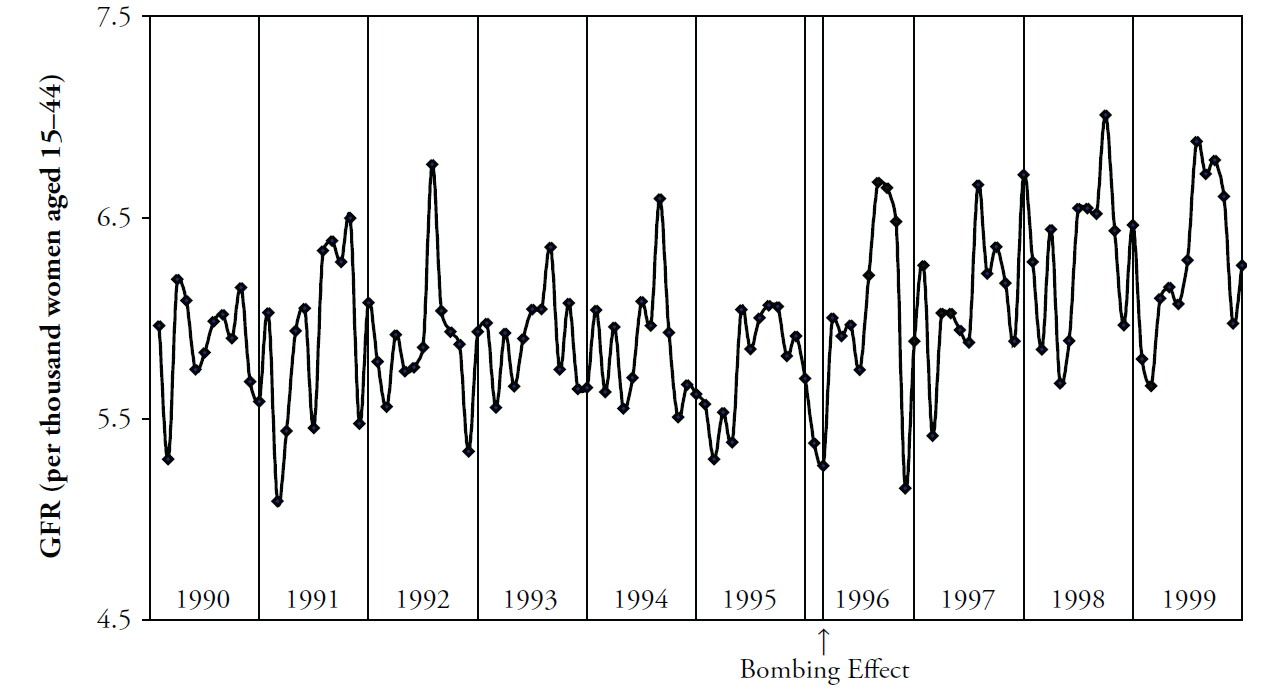## Figure 2: Cartesian Rolling - 2014 Version

Smoothed monthly birth rates (General Fertility Rates; GFRs) for Oklahoma County, 1990-1999, plotted in a linear plot. The top plot shows the connected raw data with a February smoother; the middle plot shows smoothing with a 12-month moving average, blue/green line, superimposed on a February smoother, red line); the bottom plot shows the smoothers and confidence bands, which are H-spreads defined using the distribution of GFRs for the given month and 11 previous months.

First, some R packages are loaded, and some variables and functions are defined.

change_month <- base::as.Date("1996-02-15") #as.Date("1995-04-19") + lubridate::weeks(39) = "1996-01-17"
set.seed(444) # So bootstrap won't trigger a git diff

vp_layout <- function(x, y) {
grid::viewport(layout.pos.row = x, layout.pos.col = y)
}
base::range(scores) # A new function isn't necessary.  It's defined in order to be consistent.
}
stats::quantile(x = scores, probs = c(.25, .75))
}
base::mean(scores) + base::c(-1, 1) * stats::sd(scores) / base::sqrt(base::sum(!base::is.na(scores)))
}
boot_spread <- function(scores, conf = .68) {
plugin <- function(d, i) {
base::mean(d[i])
}

distribution <- boot::boot(data = scores, plugin, R = 99) # 999 for the publication
ci <- boot::boot.ci(distribution, type = c("bca"), conf = conf)
ci$bca[4:5] # The fourth & fifth elements correspond to the lower & upper bound. } dark_theme <- ggplot2::theme( axis.title = ggplot2::element_text(color = "gray30", size = 9), axis.text.x = ggplot2::element_text(color = "gray30", hjust = 0), axis.text.y = ggplot2::element_text(color = "gray30"), axis.ticks = ggplot2::element_blank(), # panel.grid.minor.y = element_line(color = "gray95", linewidth = .1), # panel.grid.major = element_line(color = "gray90", linewidth = .1), panel.spacing = grid::unit(c(0, 0, 0, 0), "cm"), plot.margin = grid::unit(c(0, 0, 0, 0), "cm") ) # qplot(mtcars$hp) + dark_theme

light_theme <-
dark_theme +
ggplot2::theme(
axis.title          = ggplot2::element_text(color = "gray80", size = 9),
axis.text.x         = ggplot2::element_text(color = "gray80", hjust = 0),
axis.text.y         = ggplot2::element_text(color = "gray80"),
panel.grid.minor.y  = ggplot2::element_line(color = "gray99", linewidth = .1),
panel.grid.major    = ggplot2::element_line(color = "gray95", linewidth = .1)
)
date_sequence <-
base::seq.Date(
from = base::as.Date("1990-01-01"),
to   = base::as.Date("1999-01-01"),
by   = "years"
)
x_scale <-
ggplot2::scale_x_date(
breaks = date_sequence,
labels = scales::date_format("%Y")
)
# This keeps things proportional down the three frames.
x_scale_blank <-
ggplot2::scale_x_date(
breaks = date_sequence,
labels = NULL
)

### Individual Components

Here is the basic linear rolling graph. It doesn’t require much specification, and will work with a wide range of appropriate datasets. This first (unpublished) graph displays all components.

# Uncomment the next two lines to use the version built into the package.  By default, it uses the
# CSV to promote reproducible research, since the CSV format is more open and accessible to more software.

ds_linear_all <-
county_month_birth_rate_2005_version |>
tibble::as_tibble()

ds_linear_okc <-
ds_linear_all |>
dplyr::filter(county_name == "oklahoma") |>
augment_year_data_with_month_resolution(date_name = "date")

portfolio_cartesian <-
annotate_data(
ds_linear_okc,
dv_name         = "birth_rate",
center_function = stats::median,
)

cartesian_rolling(
ds_linear           = portfolio_cartesian$ds_linear, x_name = "date", y_name = "birth_rate", stage_id_name = "stage_id", change_points = change_month, change_point_labels = "Bombing Effect" )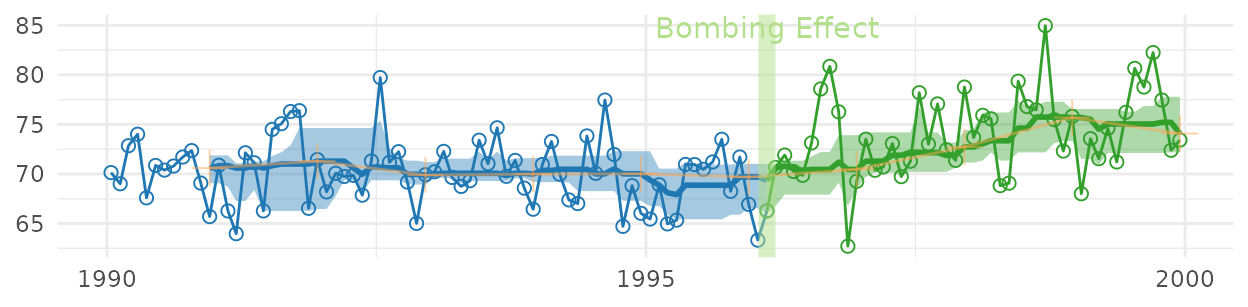The version for the manuscript was tweaked to take advantage of certain features of the dataset. This is what it looks like when all three stylized panels are combined. top_panel <- Wats::cartesian_rolling( ds_linear = portfolio_cartesian$ds_linear,
x_name                = "date",
y_name                = "birth_rate",
stage_id_name         = "stage_id",
change_points         = change_month,
y_title               = "General Fertility Rate",
change_point_labels   = "Bombing Effect",
draw_rolling_band     = FALSE,
draw_rolling_line     = FALSE
)

middle_panel <-
Wats::cartesian_rolling(
ds_linear             = portfolio_cartesian$ds_linear, x_name = "date", y_name = "birth_rate", stage_id_name = "stage_id", change_points = change_month, y_title = "General Fertility Rate", change_point_labels = "", draw_rolling_band = FALSE, draw_jagged_line = FALSE ) bottom_panel <- Wats::cartesian_rolling( ds_linear = portfolio_cartesian$ds_linear,
x_name                = "date",
y_name                = "birth_rate",
stage_id_name         = "stage_id",
change_points         = change_month,
y_title               = "General Fertility Rate",
change_point_labels   = "",
# draw_rolling_band   = FALSE,
draw_jagged_line      = FALSE
)

top_panel    <- top_panel    + x_scale       + dark_theme
middle_panel <- middle_panel + x_scale       + dark_theme
bottom_panel <- bottom_panel + x_scale_blank + dark_theme

grid::grid.newpage()
grid::pushViewport(grid::viewport(layout = grid::grid.layout(3,1)))
print(top_panel   , vp = vp_layout(1, 1))
print(middle_panel, vp = vp_layout(2, 1))
print(bottom_panel, vp = vp_layout(3, 1))
grid::popViewport()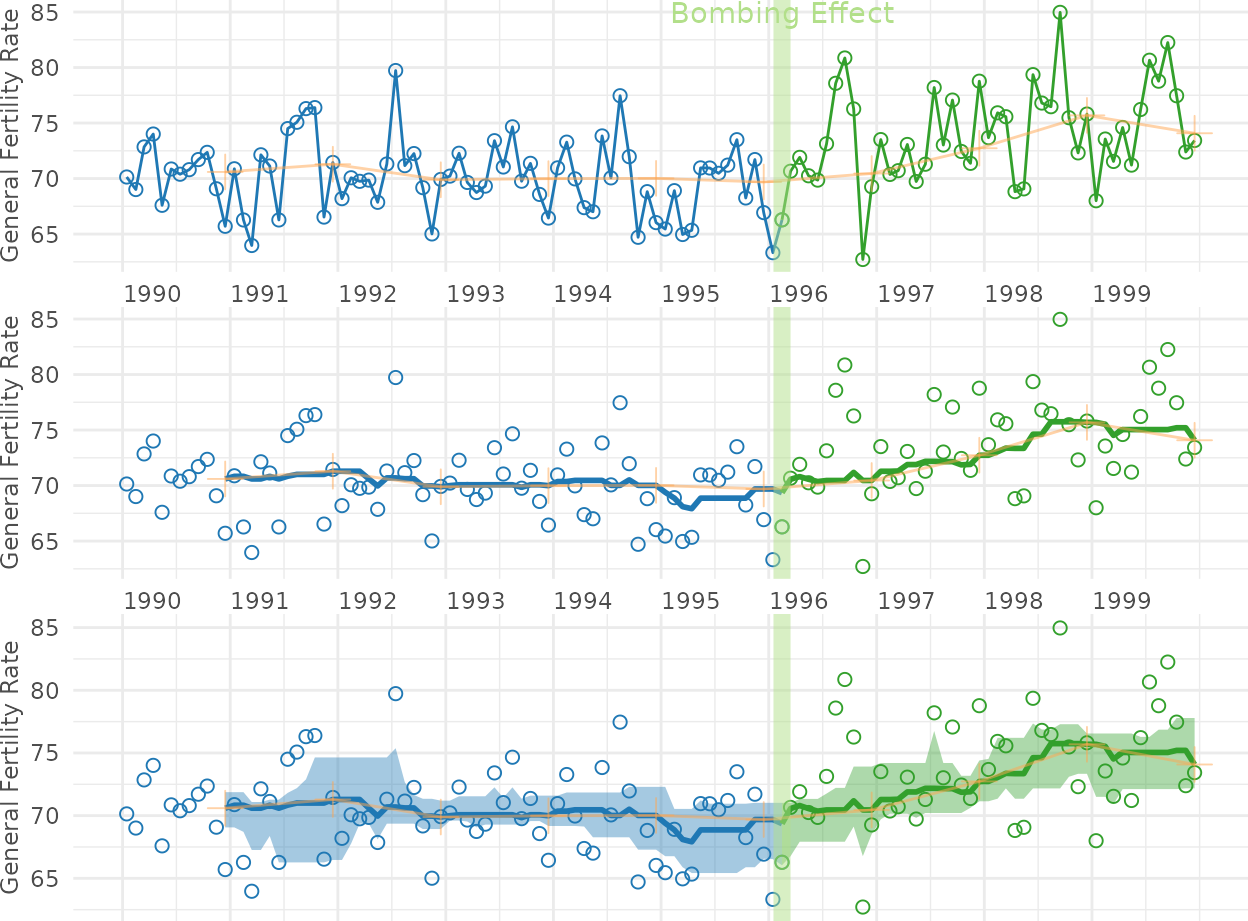## Figure 4: Cartesian Periodic

Cartesian plot of the GFR time series data in Oklahoma County, with H-spread Bands superimposed.

cartesian_periodic <-
Wats::cartesian_periodic(
portfolio_cartesian$ds_linear, portfolio_cartesian$ds_periodic,
x_name                  = "date",
y_name                  = "birth_rate",
stage_id_name           = "stage_id",
change_points           = change_month,
change_point_labels     = "Bombing Effect",
y_title                 = "General Fertility Rate",
draw_periodic_band      = TRUE #The only difference from the simple linear graph above
)
print(cartesian_periodic)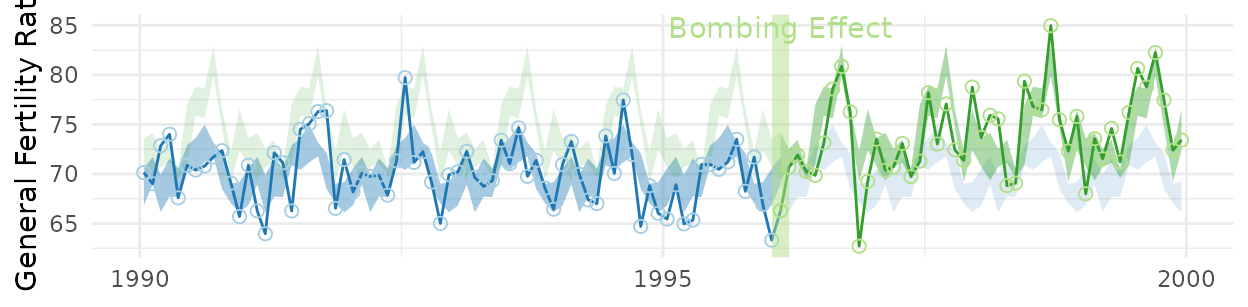cartesian_periodic <- cartesian_periodic + x_scale + dark_theme
print(cartesian_periodic)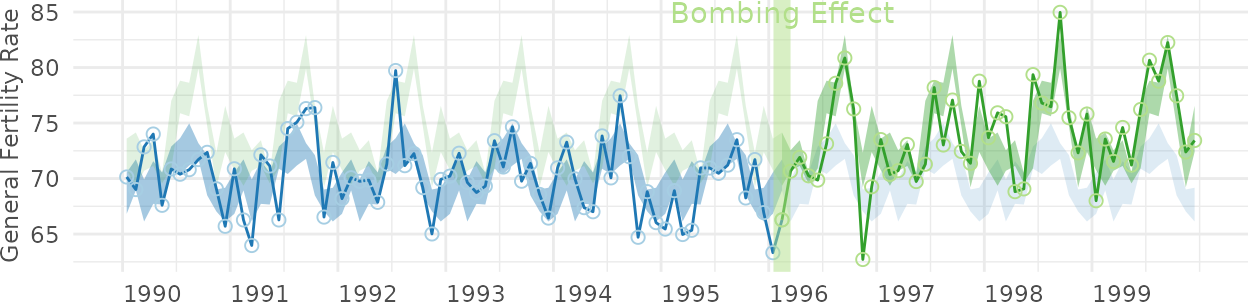## Figure 5: Polar Periodic

Wrap Around Time Series (WATS Plot) of the Oklahoma City GFR data, 1990-1999.

portfolio_polar <-
polarize_cartesian(
ds_linear                       = portfolio_cartesian$ds_linear, ds_stage_cycle = portfolio_cartesian$ds_stage_cycle,
y_name                          = "birth_rate",
stage_id_name                   = "stage_id",
plotted_point_count_per_cycle   = 7200
)

grid::grid.newpage()
polar_periodic(
ds_linear             = portfolio_polar$ds_observed_polar, ds_stage_cycle = portfolio_polar$ds_stage_cycle_polar,
stage_id_name         = "stage_id",
draw_periodic_band    = FALSE,
draw_stage_labels     = TRUE,
cardinal_labels       = c("Jan1", "Apr1", "July1", "Oct1")
)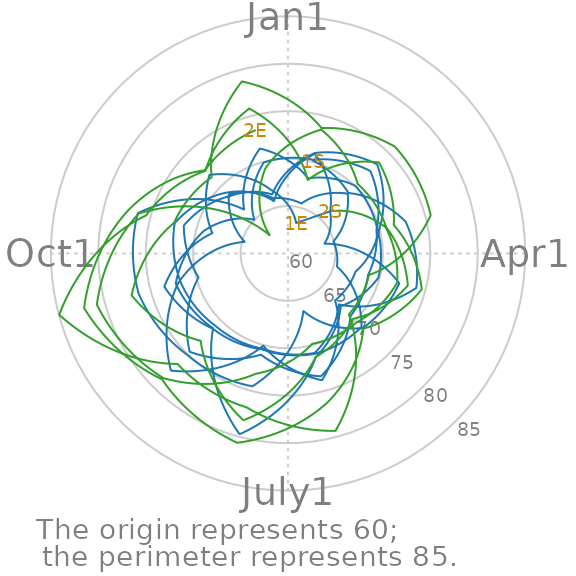## Figure 6: WATS and Cartesian

Wrap Around Time Series (WATS Plot) of the Oklahoma City GFR data, 1990-1999.

portfolio_polar <-
Wats::polarize_cartesian(
ds_linear                     = portfolio_cartesian$ds_linear, ds_stage_cycle = portfolio_cartesian$ds_stage_cycle,
y_name                        = "birth_rate",
stage_id_name                 = "stage_id",
plotted_point_count_per_cycle = 7200
)

grid::grid.newpage()
grid::pushViewport(grid::viewport(
layout = grid::grid.layout(
nrow    = 2,
ncol    = 2,
respect = TRUE,
widths  = grid::unit(c(1,  1), c("null", "null")),
heights = grid::unit(c(1, .5), c("null", "null"))
),
gp = grid::gpar(cex = 1, fill = NA)
))

## Create top left panel
grid::pushViewport(grid::viewport(layout.pos.col = 1, layout.pos.row = 1))
top_left_panel <-
Wats::polar_periodic(
ds_linear             = portfolio_polar$ds_observed_polar, ds_stage_cycle_polar = portfolio_polar$ds_stage_cycle_polar,
stage_id_name         = "stage_id", #graph_ceiling = 7,
cardinal_labels       = c("Jan1", "Apr1", "July1", "Oct1")
)
grid::upViewport()

## Create top right panel
grid::pushViewport(grid::viewport(layout.pos.col = 2, layout.pos.row = 1))
top_right_panel <-
Wats::polar_periodic(
ds_linear             = portfolio_polar$ds_observed_polar, ds_stage_cycle_polar = portfolio_polar$ds_stage_cycle_polar,
stage_id_name         = "stage_id", #graph_ceiling = 7,
draw_observed_line    = FALSE,
cardinal_labels       = c("Jan1", "Apr1", "July1", "Oct1"),
origin_label          = NULL
)
grid::upViewport()

## Create bottom panel
grid::pushViewport(grid::viewport(layout.pos.col = 1:2, layout.pos.row = 2, gp = grid::gpar(cex = 1)))
print(cartesian_periodic, vp = vp_layout(x = 1:2, y = 2)) # Print across both columns of the bottom row.
grid::upViewport()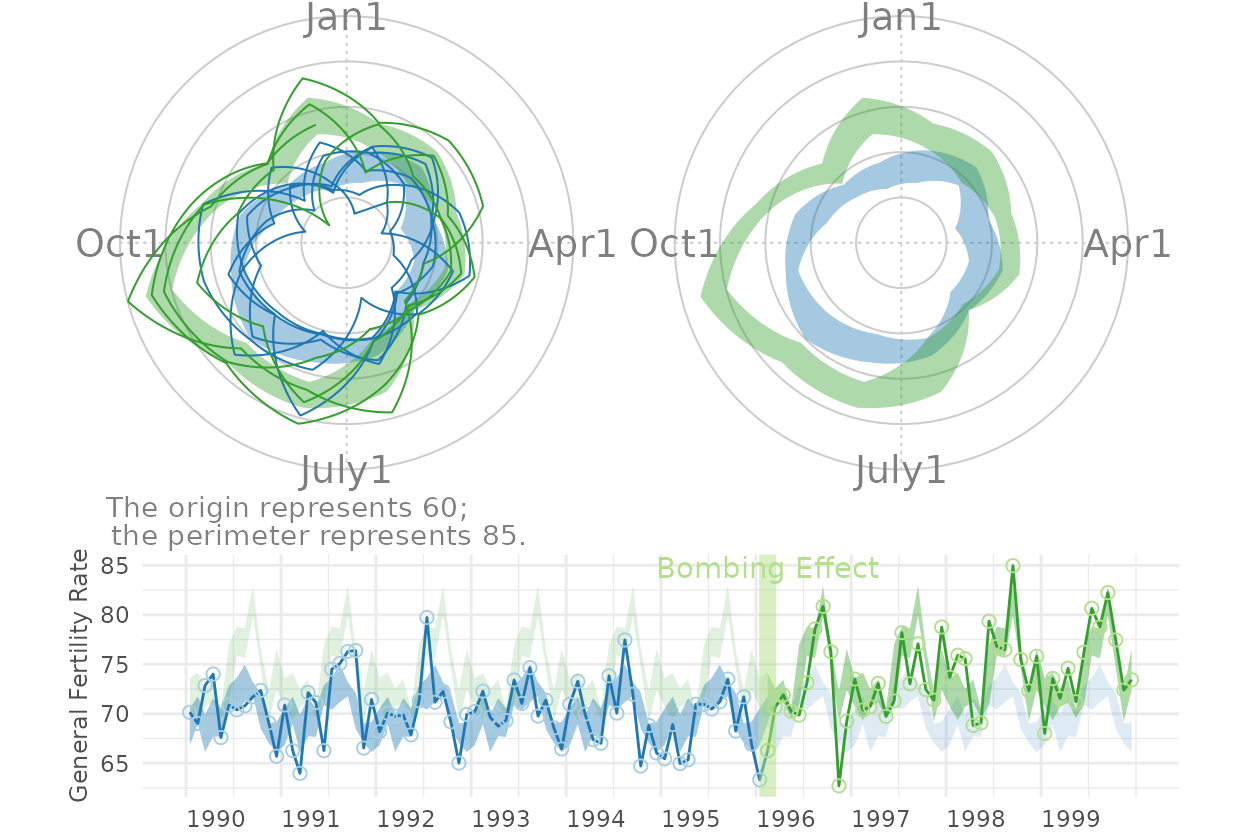## Figure 7: County Comparison

This figure compares Oklahoma County against the (other) largest urban counties.

# ds_linear_all <- Wats::augment_year_data_with_month_resolution(ds_linear = county_month_birth_rate_2005_version, date_name="date")

# Identify the average size of the fecund population
ds_linear_all |>
dplyr::group_by(county_name) |>
dplyr::summarize(
Mean = base::mean(fecund_population)
) |>
dplyr::ungroup()
 [38;5;246m# A tibble: 12 × 2 [39m
county_name     Mean
[3m [38;5;246m<chr> [39m [23m           [3m [38;5;246m<dbl> [39m [23m
[38;5;250m 1 [39m canadian       [4m1 [24m [4m8 [24m332.
[38;5;250m 2 [39m cleveland      [4m4 [24m [4m8 [24m865.
[38;5;250m 3 [39m comanche       [4m2 [24m [4m6 [24m268.
[38;5;250m 4 [39m creek          [4m1 [24m [4m3 [24m402.
[38;5;250m 5 [39m logan           [4m7 [24m065.
[38;5;250m 6 [39m mcclain         [4m5 [24m434.
[38;5;250m 7 [39m oklahoma      [4m1 [24m [4m4 [24m [4m6 [24m882.
[38;5;250m 8 [39m osage           [4m8 [24m529.
[38;5;250m 9 [39m pottawatomie   [4m1 [24m [4m3 [24m604.
[38;5;250m10 [39m rogers         [4m1 [24m [4m3 [24m383.
[38;5;250m11 [39m tulsa         [4m1 [24m [4m2 [24m [4m3 [24m783.
[38;5;250m12 [39m wagoner        [4m1 [24m [4m1 [24m580.
graph_row_comparison <- function(
row_label       = "",
.county_name    = "oklahoma",
change_month    = as.Date("1996-02-15")
) {
ds_linear <-
ds_linear_all |>
dplyr::filter(county_name == .county_name) |>
Wats::augment_year_data_with_month_resolution(date_name = "date")

portfolio_cartesian <-
ds_linear |>
Wats::annotate_data(
dv_name         = "birth_rate",
center_function = stats::median,
)

portfolio_polar <-
portfolio_cartesian$ds_linear |> Wats::polarize_cartesian( ds_stage_cycle = portfolio_cartesian$ds_stage_cycle,
y_name                        = "birth_rate",
stage_id_name                 = "stage_id",
plotted_point_count_per_cycle = 7200
)

cartesian_periodic <-
portfolio_cartesian$ds_linear |> Wats::cartesian_periodic( portfolio_cartesian$ds_periodic,
x_name                            = "date",
y_name                            = "birth_rate",
stage_id_name                     = "stage_id",
change_points                     = change_month,
change_point_labels               = ""
)

grid::pushViewport(grid::viewport(
layout =
grid::grid.layout(
nrow    = 1,
ncol    = 3,
respect = FALSE,
widths  = grid::unit(c(1.5, 1, 3), c("line", "null", "null"))
),
gp = grid::gpar(cex = 1, fill = NA)
))

grid::pushViewport(grid::viewport(layout.pos.col = 1))
grid::grid.rect(gp = grid::gpar(fill = "gray90", col = NA))
grid::grid.text(row_label, rot = 90)
grid::popViewport()

grid::pushViewport(grid::viewport(layout.pos.col = 2))
Wats::polar_periodic(
ds_linear               = portfolio_polar$ds_observed_polar, ds_stage_cycle_polar = portfolio_polar$ds_stage_cycle_polar,
draw_observed_line      = FALSE,
stage_id_name           = "stage_id",
origin_label            = NULL,
plot_margins            = c(0, 0, 0, 0)
)
grid::popViewport()

grid::pushViewport(grid::viewport(layout.pos.col = 3))
print(cartesian_periodic + x_scale + light_theme, vp = vp_layout(x = 1, y = 1))
grid::popViewport()
grid::popViewport() #Finish the row
}

county_names  <- c("Comanche", "Cleveland", "Oklahoma", "Tulsa", "Rogers")
counties      <- tolower(county_names)

grid::grid.newpage()
grid::pushViewport(grid::viewport(
layout  = grid::grid.layout(nrow = length(counties), ncol = 1),
gp      = grid::gpar(cex = 1, fill = NA)
))

for (i in base::seq_along(counties)) {
grid::pushViewport(grid::viewport(layout.pos.row = i))
graph_row_comparison(.county_name = counties[i], row_label = county_names[i])
grid::popViewport()
}
grid::popViewport()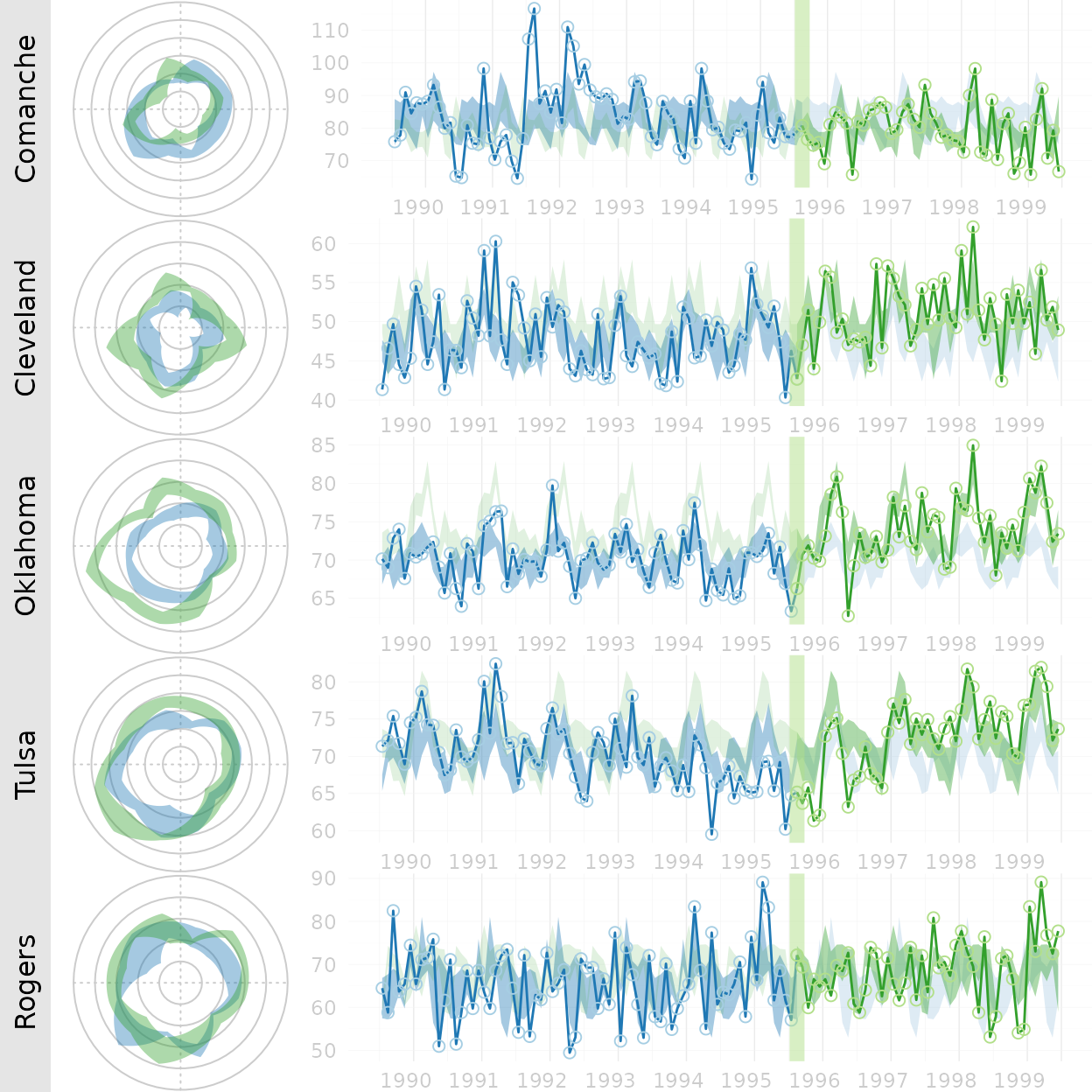Here are all 12 counties that Ronnie collected birth records for. This extended graph is not in the manuscript.

counties <- base::sort(base::unique(ds_linear_all\$county_name))
county_names <- c("Canadian", "Cleveland", "Comanche", "Creek", "Logan", "McClain", "Oklahoma", "Osage", "Pottawatomie", "Rogers", "Tulsa", "Wagoner")

grid::grid.newpage()
grid::pushViewport(grid::viewport(
layout  = grid::grid.layout(nrow = base::length(counties), ncol = 1),
gp      = grid::gpar(cex = 1, fill = NA)
))

for (i in base::seq_along(counties)) {
grid::pushViewport(grid::viewport(layout.pos.row = i))
graph_row_comparison(.county_name = counties[i], row_label = county_names[i])
grid::popViewport()
}
grid::popViewport()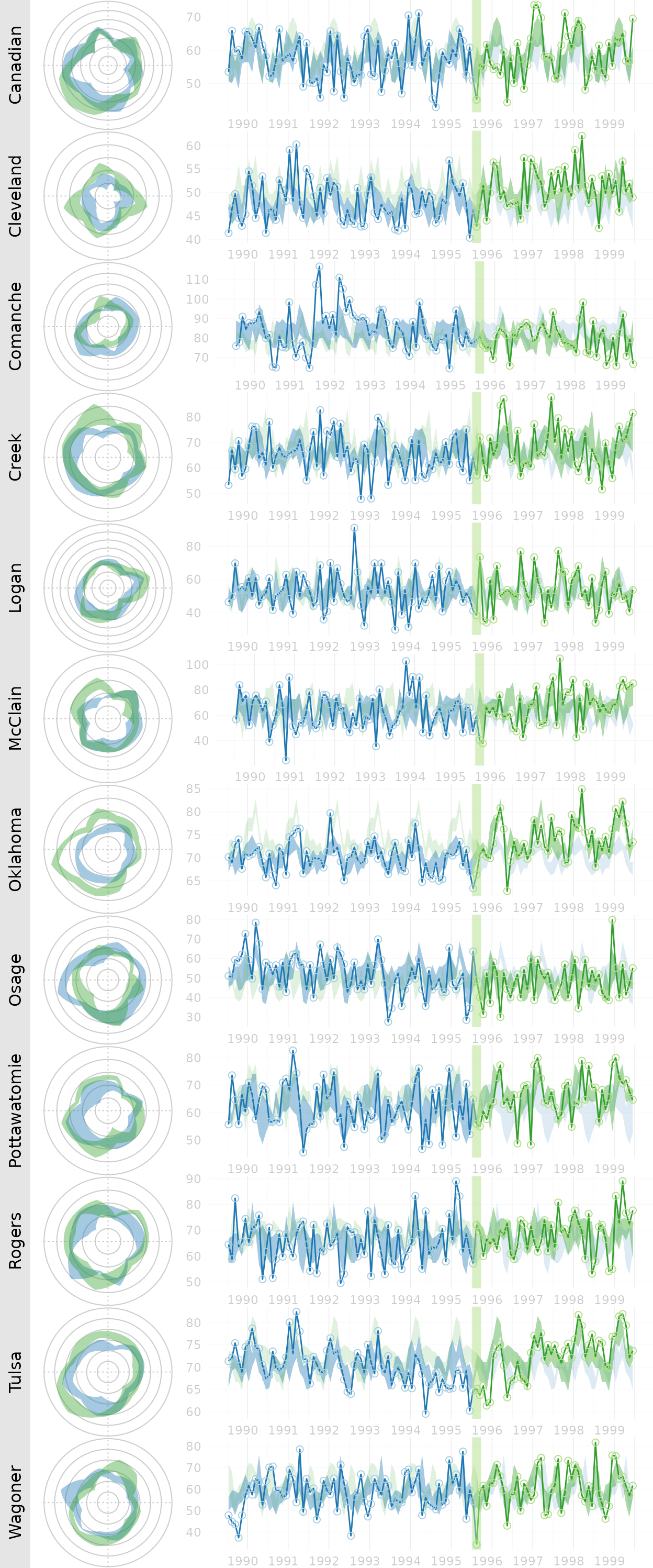## Figure 8: Error Band Comparison

This figure demonstrates that WATS accommodates many types of error bands.

spreads <- c("h_spread", "full_spread", "se_spread", "boot_spread")
grid::grid.newpage()
grid::pushViewport(grid::viewport(
layout  = grid::grid.layout(nrow = base::length(spreads), ncol = 1),
gp      = grid::gpar(cex = 1, fill = NA)
))
grid::pushViewport(grid::viewport(layout.pos.row = i))
grid::upViewport()
}
grid::upViewport()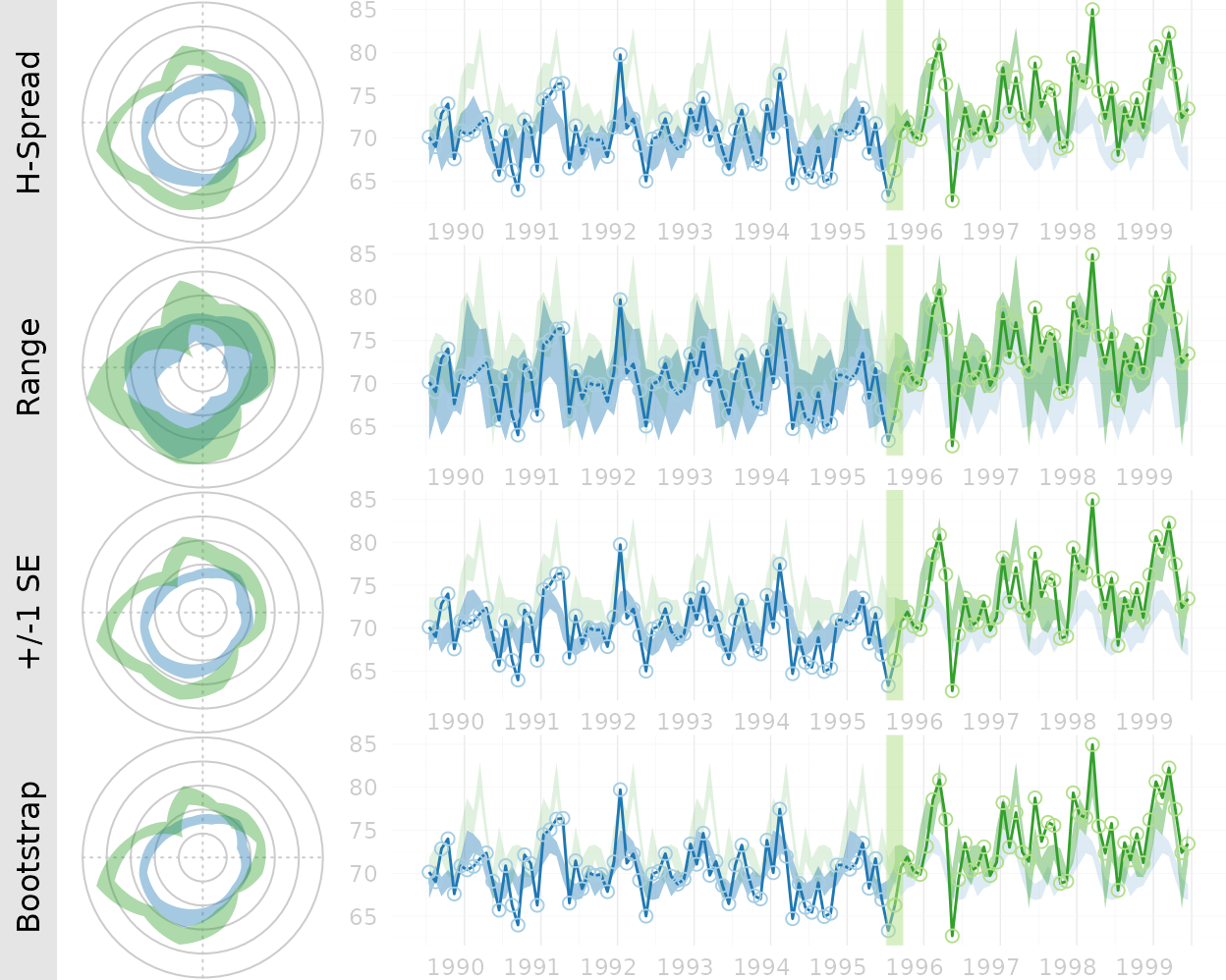## Session Info

The current vignette was build on a system using the following software.

Report created by runner at Mon Sep 11 13:55:41 2023, +0000
R version 4.3.1 (2023-06-16)
Platform: x86_64-pc-linux-gnu (64-bit)
Running under: Ubuntu 22.04.3 LTS

Matrix products: default

locale:
 LC_CTYPE=C.UTF-8       LC_NUMERIC=C           LC_TIME=C.UTF-8
 LC_COLLATE=C.UTF-8     LC_MONETARY=C.UTF-8    LC_MESSAGES=C.UTF-8
 LC_TELEPHONE=C         LC_MEASUREMENT=C.UTF-8 LC_IDENTIFICATION=C

time zone: UTC
tzcode source: system (glibc)

attached base packages:
 stats     graphics  grDevices utils     datasets  methods   base

other attached packages:
 Wats_1.0.1.9000

loaded via a namespace (and not attached):
 sass_0.4.7         utf8_1.2.3         generics_0.1.3     stringi_1.7.12
 lattice_0.21-8     digest_0.6.33      magrittr_2.0.3     RColorBrewer_1.1-3
 evaluate_0.21      grid_4.3.1         timechange_0.2.0   fastmap_1.1.1
 rprojroot_2.0.3    jsonlite_1.8.7     purrr_1.0.2        fansi_1.0.4
 scales_1.2.1       textshaping_0.3.6  jquerylib_0.1.4    cli_3.6.1
 rlang_1.1.1        munsell_0.5.0      withr_2.5.0        cachem_1.0.8
 yaml_2.3.7         tools_4.3.1        memoise_2.0.1      dplyr_1.1.3
 colorspace_2.1-0   ggplot2_3.4.3      boot_1.3-28.1      vctrs_0.6.3
 R6_2.5.1           zoo_1.8-12         lifecycle_1.0.3    lubridate_1.9.2
 testit_0.13        stringr_1.5.0      fs_1.6.3           ragg_1.2.5
 pkgconfig_2.0.3    desc_1.4.2         pkgdown_2.0.7      pillar_1.9.0
 bslib_0.5.1        gtable_0.3.4       glue_1.6.2         systemfonts_1.0.4
 highr_0.10         xfun_0.40          tibble_3.2.1       tidyselect_1.2.0
 knitr_1.43         farver_2.1.1       htmltools_0.5.6    labeling_0.4.3
 rmarkdown_2.24     compiler_4.3.1